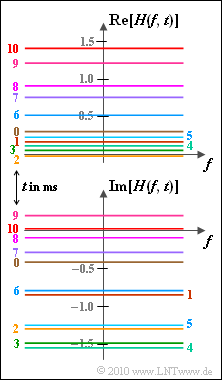# Exercise 2.1Z: 2D-Frequency and 2D-Time Representations2D transfer function:
real and imaginary parts

To describe a time-variant channel with several paths, the  two-dimensional impulse response  is used:

$$h(\tau,\hspace{0.05cm}t) = \sum_{m = 1}^{M} z_m(t) \cdot {\rm \delta} (\tau - \tau_m)\hspace{0.05cm}.$$

The first parameter  $(\tau)$  indicates the delay, the second parameter $(t)$  is related to the time variance of the channel.

The Fourier transform of  $h(\tau, \ t)$  with respect to  $\tau$  is the  time-variant transfer function:

$$H(f,\hspace{0.05cm} t) \hspace{0.2cm} \stackrel {f,\hspace{0.05cm}\tau}{\bullet\!\!-\!\!\!-\!\!\!-\!\!\circ} \hspace{0.2cm} h(\tau,\hspace{0.05cm}t) \hspace{0.05cm}.$$
• In the graph,  $H(f, \ t)$  is displayed as a function of frequency, for different values of absolute time  $t$  in the range of  $0 \ \text{...} \ 10 \ \rm ms$.
• In general,  $H(f, \ t)$  is complex.  The real part (top) and the imaginary part (bottom) are drawn separately.

Notes:

• This task belongs to the chapter  General description of time–variant systems.
• In the above equation, an single-path channel is represented with parameter  $M = 1$ .
• Here are some numerical values of the specified time-variant transfer function:
$$H(f,\hspace{0.05cm} t \hspace{-0.1cm} \ = \ \hspace{-0.1cm} 0\, {\rm ms}) \approx 0.3 - {\rm j} \cdot 0.4 \hspace{0.05cm},\hspace{0.2cm} H(f,\hspace{0.05cm} t = 2\, {\rm ms}) \approx 0.0 - {\rm j} \cdot 1.3 \hspace{0.05cm},$$
$$H(f,\hspace{0.05cm} t \hspace{-0.1cm} \ = \ \hspace{-0.1cm} 4\, {\rm ms}) \approx 0.1 - {\rm j} \cdot 1.5 \hspace{0.05cm},\hspace{0.2cm} H(f,\hspace{0.05cm} t = 6\, {\rm ms}) \approx 0.5 - {\rm j} \cdot 0.8 \hspace{0.05cm},$$
$$H(f,\hspace{0.05cm} t \hspace{-0.1cm} \ = \ \hspace{-0.1cm} 8\, {\rm ms}) \approx 0.9 - {\rm j} \cdot 0.1 \hspace{0.05cm},\hspace{0.2cm} H(f,\hspace{0.05cm} t = 10\, {\rm ms}) \approx 1.4 \hspace{0.05cm}.$$
• As can already be guessed from the above graph, neither the real nor the imaginary part of the 2D transfer function  $H(f, \ t)$  are zero-mean.

### Questionnaire

1

Is the channel time-variant?

 Yes. No.

2

Is it a multi-path channel?

 Yes. No.

3

How can the 2D impulse response be described here?

 $h(\tau, \ t) = A \cdot \delta(\tau) + B \cdot \delta(\tau \, –5 \, \rm µ s)$. $h(\tau, \ t) = A \cdot \delta(\tau)$. $h(\tau, \ t) = z(t) \cdot \delta(\tau)$.

4

Estimate for which channel the data was recorded.

 AWGN channel, Two-way channel, Rayleigh channel, Rice channel.

### Solution

#### Solution

(1)  As can be seen in the graph, the transfer function  $H(f, \ t)$  depends on  $t$.  Thus  $h(\tau, \ t)$  is also time-dependent.  Correct is therefore YES.

(2)  If we look at a fixed point in time, for example  $t = 2 \ \rm ms$, we obtain the following for the time-variant transfer function:

$$H(f,\hspace{0.05cm} t = 2\, {\rm ms}) = - {\rm j} \cdot 1.3 \hspace{0.05cm} = {\rm const.}$$
• Thus the corresponding 2D–impulse response is
$$h(\tau,\hspace{0.05cm} t = 2\, {\rm ms}) = - {\rm j} \cdot 1.3 \cdot \delta (\tau) \hspace{0.05cm} \hspace{0.3cm}\Rightarrow \hspace{0.3cm} M = 1 \hspace{0.05cm}.$$
• There is only one path  $(M=1)$.  This means that the correct solution is NO.

(3)  The correct solution is solution 3:

• There is time variance but no frequency selectivity.
• Options 1 and 2, on the other hand, describe time-invariant systems.

(4)  Solution 4 is correct:

• For the AWGN channel, no transfer function can be specified.
• For a two-way channel,  $H(f, \ t)$  is not a constant in  $f$  for any  $t$.
• Since in the  $H(f, \ t)$  graph the real and imaginary part have a non-zero mean   ⇒   the Rayleigh–channel can also be excluded.
• The data for the present task comes from a  Rice channel  with following parameters:
$$\sigma = {1}/{\sqrt{2}} \hspace{0.05cm},\hspace{0.2cm} x_0 = {1}/{\sqrt{2}} \hspace{0.05cm},\hspace{0.2cm}y_0 = -{1}/{\sqrt{2}} \hspace{0.05cm},\hspace{0.2cm} f_{\rm D,\hspace{0.05cm} max} = 100\,\,{\rm Hz}\hspace{0.05cm}.$$